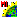# VerticesInLines.sml

###Download

#### Syntax Highlighing:

```comments, key words, predefined symbols, class members & methods, functions & classes
```
```# Script demonstrates a way to print out coordinates of line vertices
# Lists all vertices for every line in a vector object
# Be sure to first resample (warp) your vector to the Coordinate Reference System you want the vertices to be in
# Cindy Robbins - 28 April 2005
clear();
numeric i, numLines, j, numPoints, lineID, k;
class VECTOR V;
GetInputVector(V);
# Here's where the output file will go:
class FILE myFile = fopen("c:/vertices.csv");
# the following arrays will be resized automatically
array lineArray;
array xArray;
array yArray;
array zArray;
numLines = NumVectorLines(V);
for j = 1 to numLines {
numPoints = GetVectorLinePointList(V, xArray, yArray, j, zArray);
lineID = V.line[j].Internal.ElemNum;
for k = 1 to numPoints {
# modify this to format the output the way you want
fprintf(myFile, "%d%s%f%s%f%s%f\n", j, ",",xArray[k], ",", yArray[k], ",",zArray[k]);
}
}
print("Done");```